Semiconductor Laboratory

## KSV CAM 200 Optical Contact Angle Meter

The KSV CAM 200 Optical Contact Angle Meter measures the contact angle of liquid droplets on a surface. This can be used to determine surface and interface tensions.

### Static contact angle measurement

Creation of a Droplet
1. Watch the video above.
2. Turn on the device (height of sample holder and syringe are already set and focus of the lens is adjusted)
3. Place the sample on the sample holder by using tweezers
4. Open the program "CAM2008" on the computer
5. Click on the leftmost symbol
6. Give your measurement a name (leave all other parameters as they are) and press "Start"
7. Create a droplet by turning the upper part of the syringe (droplet should be between the two green lines → same volume)
8. Lower the syringe to the sample until the droplet touches the surface
9. Quickly lift up the syringe and press "Record" immediately
10. Shift the sample holder to right/left and repeat the process.
Analysis of a static contact angle measuement
1. If all wanted droplets are made and recorded, click "Done" → new window opens
2. Set a baseline (red line) at the surface
3. Add the same baseline for all records (if you did not change the height of the sample holder) by pressing "Copy Baseline to All"
4. Place the blue rectangle such, that it only contains the droplet and the white background (syringe, surface (impurities) or neighboring drops should not be within the rectangle)
5. Click "Execute"
6. Note the values of the right and the left angle (and of the volume), because they will NOT be saved!
7. Repeat this for all droplets (use the arrow button to switch between the droplets)
8. Click "Close" to close the program

### Dynamical contact angle measurement

Creation of a Droplet
1. Do the first six steps of the creation of a droplet as above.
2. Create a droplet by turning the upper part of the syringe (droplet should be between the two green lines → same volume).
3. Lower the syringe to the sample until the droplet touches the surface and then a little bit further, so the droplet sits comfortably on the surface, and press "Record".
4.5. Turn the upper part of the syringe slightly to allow the droplet to gain more volume and press "Record".
6. Repeat that process until a significant shift of the two contact points (see arrows) of the droplet is noticeable.
7.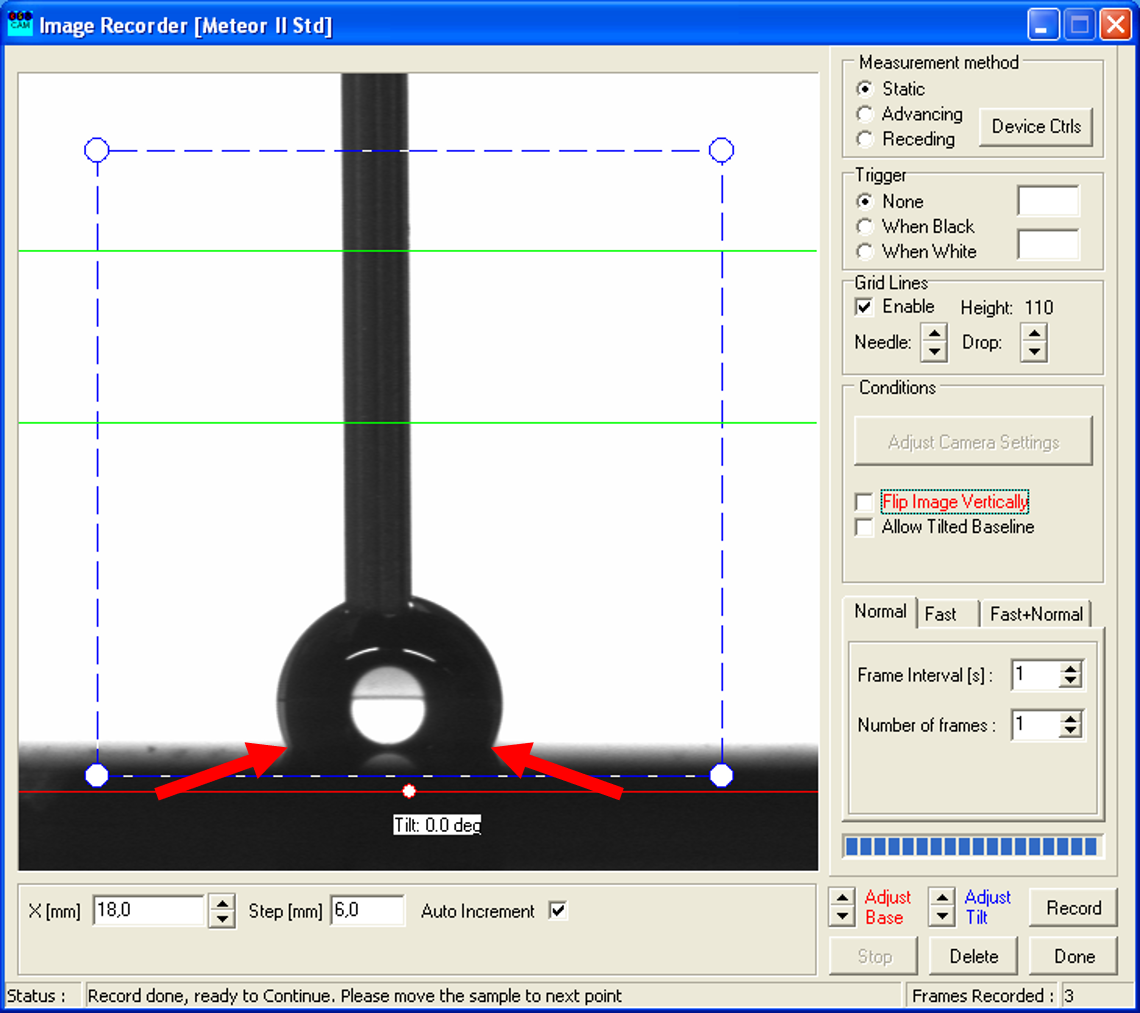8. Then turn the upper part of the syringe slightly in the other direction to decrease the volume of the droplet and press "Record". As you can see, this changes the form of the droplet.
9.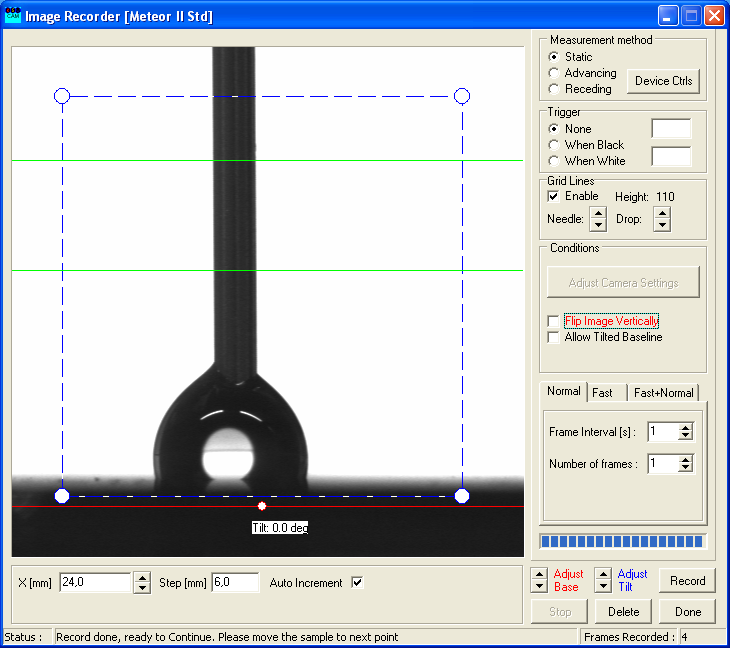10. Repeat that process until the droplet is gone or no longer in contact with the syringe.
11. Shift the sample holder to right/left and repeat the process.
Analysis
1. First three steps are the same as in the static CAM.
2. However, for the dynamic measurement of the advancing contact angle you need to go through the pictures taken while increasing the volume of the droplet and find the ones where the two contact points shift significantly.
3.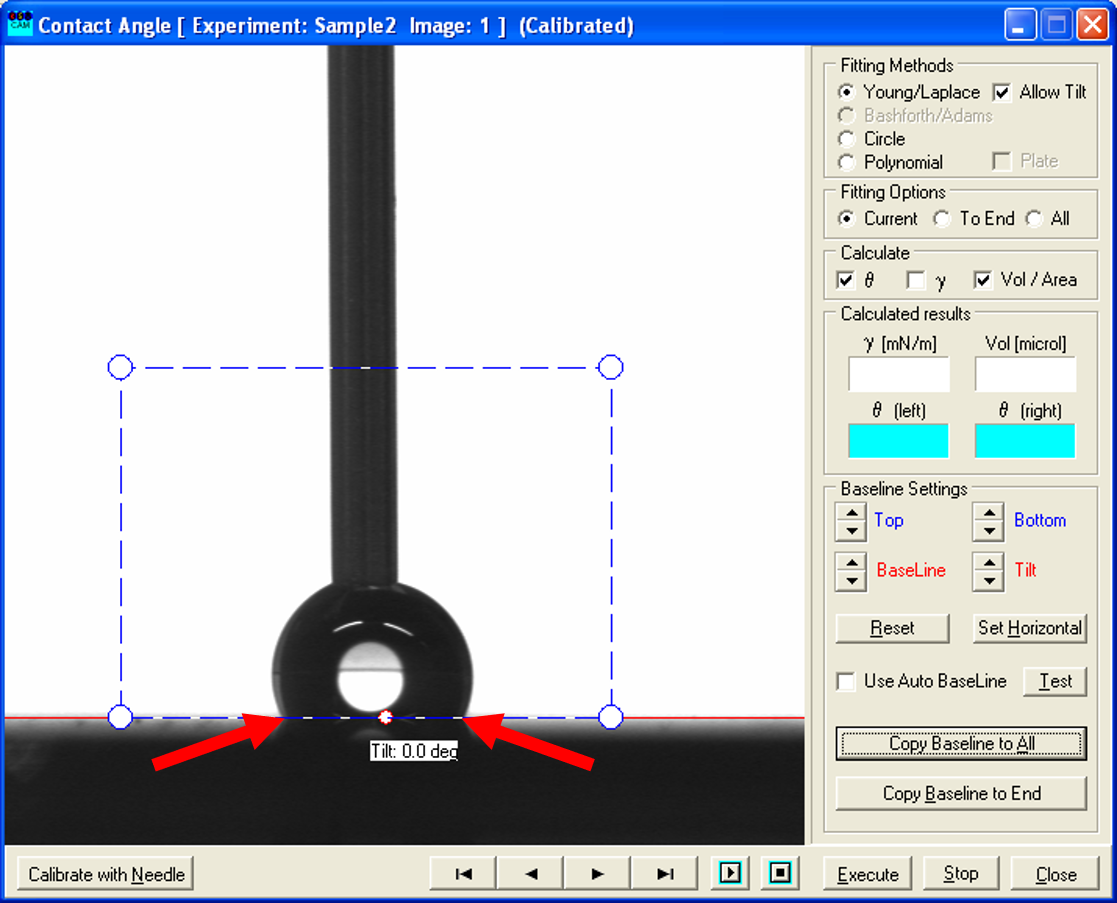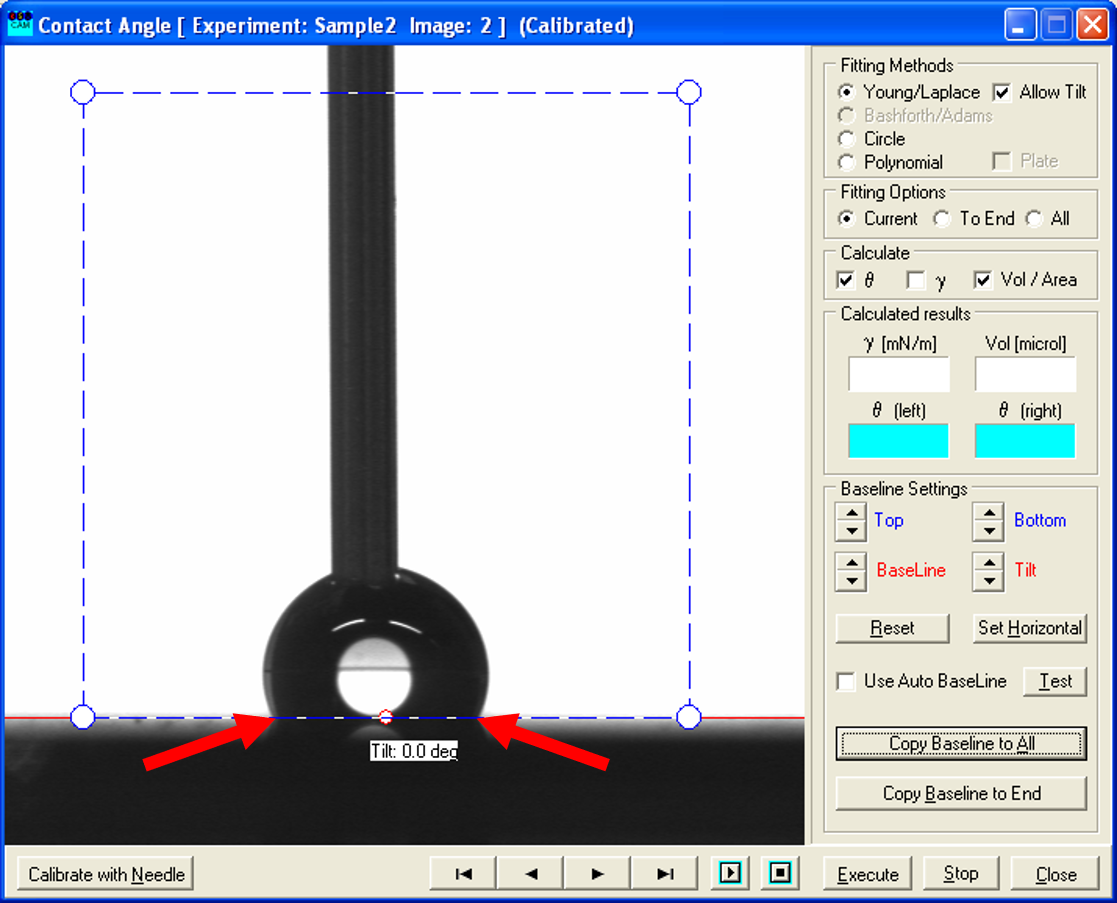4. Use the recorded picture before the shift (left one) and place the blue rectangle such, that it only contains the droplet and the white background as before.
5. Click "Execute" and note the values of the right and the left angle (and of the volume), because they will NOT be saved! This gives you the advancing contact angle.
6. For the receding contact angle, the process is similar: here you look through the pictures taken while decreasing the volume of the drop and determine the ones where the contact points shift significantly closer and the shape of the droplet possible changes.
7.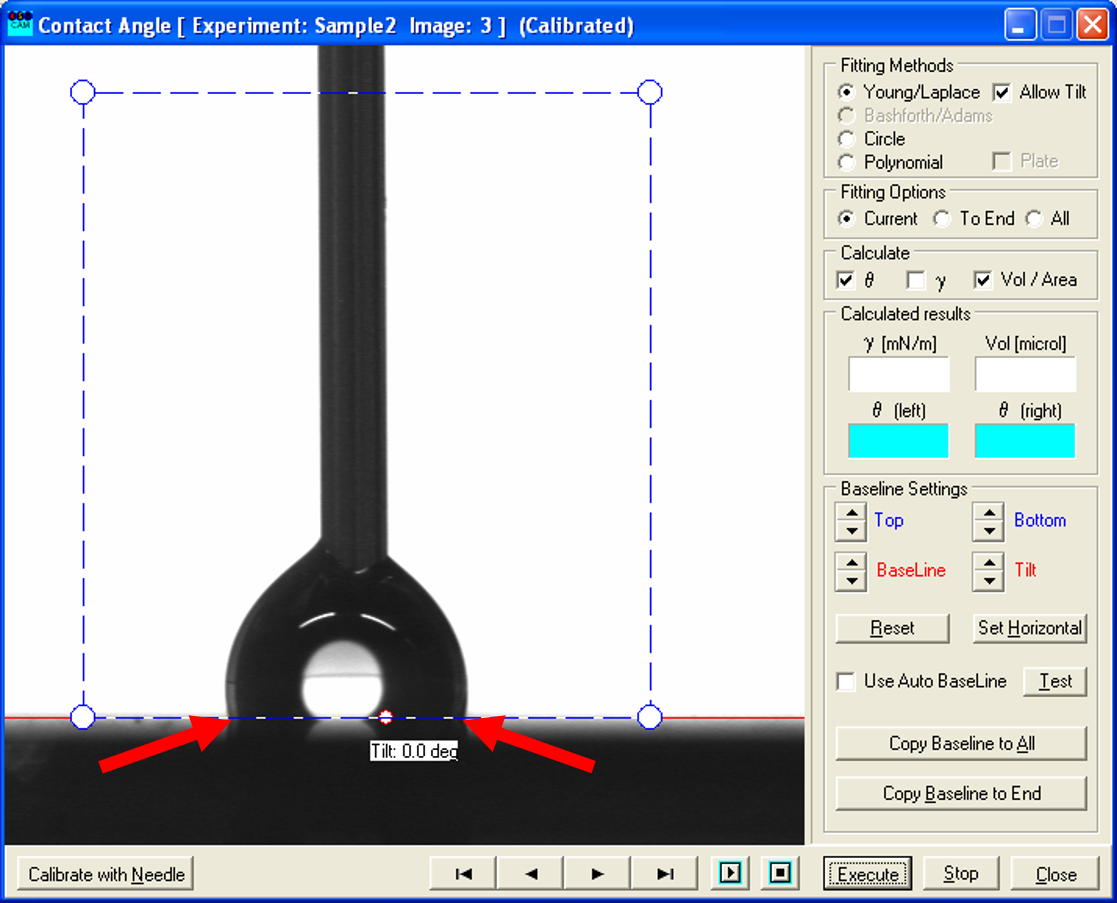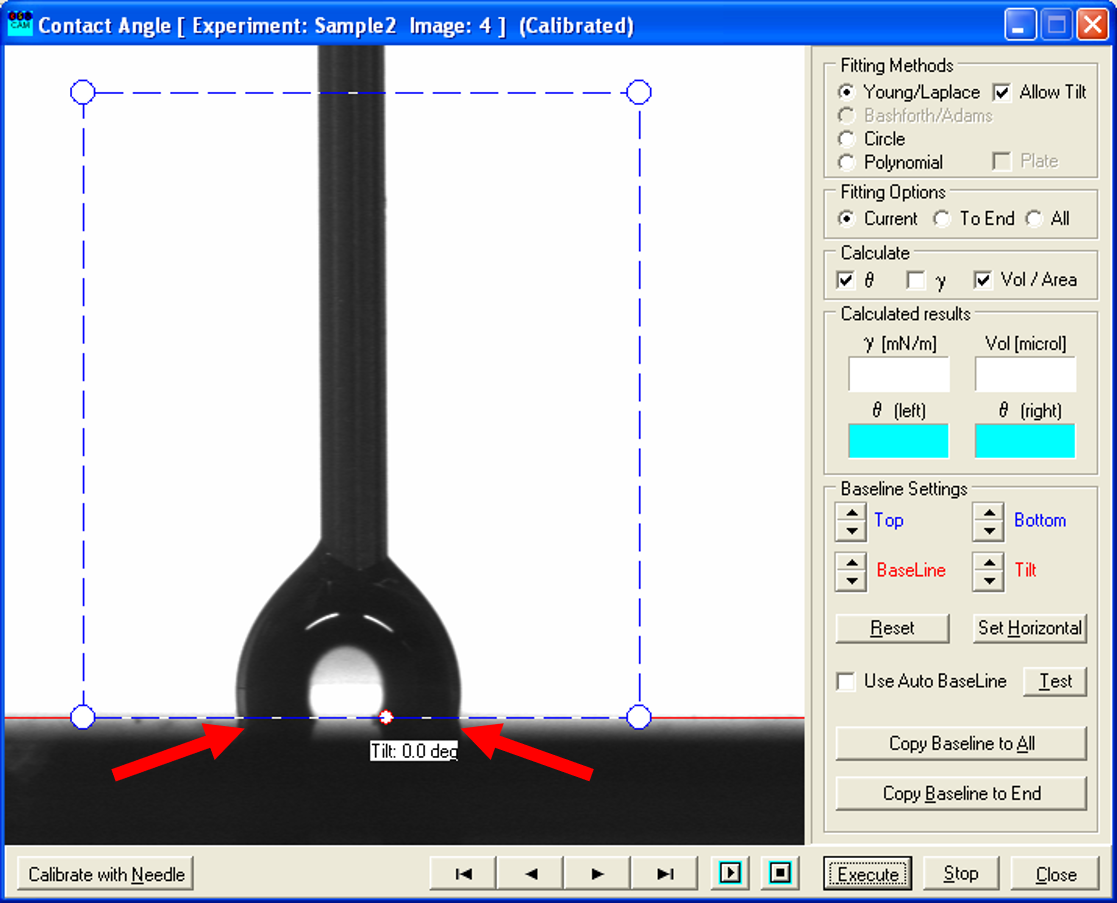8. Again, use the recorded picture before the shift (left one) and measure the contact angle as described above. This constitutes the receding contact angle.
9. Repeat this for all droplets you have recorded.
10. Click "Close" to close the program.

### Introduction

Water contact angle (WCA) is a technique to determine wettability, hydrophobicity, and the strength of contact in a solid-liquid interaction. It is a straightforward technique where a water drop is cast onto the surface of the sample. Water balls up on a hydrophobic surface but it flattens out on a hydrophillic surface.

### Theory

Many phenomena that are observed in systems of interfaces in which one of the phases is liquid can be described by the concept of surface tension $(\gamma )$. For example, that drops of liquid tend to be spherical or the observation that water spreads evenly on some surfaces, while remaining in isolated drops on others.

Due to the surface tension the area of an interface tends to a minimum, therefore a drop of water tends to assume a spherical shape. In a molecular point of view, the forces acting on a molecule at the surface of a liquid are compared to those acting on one in the bulk. The attractive forces on a molecule in the bulk are isotropic, when averaged over time. This means that there is no force which pulls the molecule in any direction. Due to missing neighbors in the direction of the gas phase, the force is unbalanced for surface molecules and therefore the surface molecules are pulled inside the bulk.

If a drop of liquid is placed onto a solid surface, the droplet is in equilibrium by balancing three forces, namely, the interfacial tensions, between solid and liquid $(\gamma_{LS})$, that between solid and vapor $(\gamma_{SV})$ and that between liquid and vapor $(\gamma_{LV})$. At the three-phase boundary where liquid, gas and vapor interacts the contact angle $\theta_c$ is formed as shown in the figure.Contact angle forces. Wikimedia

Equation (1) describes a droplet in equilibrium when all three forces are balanced:

$$\gamma_{SV}=\gamma_{LS}+\gamma_{LV}\cos\theta_c\qquad(1)$$

If $\gamma_{SV}$ is smaller than $\gamma_{LS}$, $\cos \theta_c$ will be negative, and therefore $\theta_c$ is smaller than 90° (the droplet wets). This occurs with a high surface-energy solid (metal), or a low surface-tension liquid. If $\gamma_{SV}$ is larger than $\gamma_{SLS}$, $\cos \theta_c$ will be positive, and therefore $\theta_c$ is bigger than 90° (the droplet dewets). This occurs with a low surface-energy solid, or a high surface-tension liquid (water). 

For a better understanding of the origin of contact angles one has to consider the balance of forces between molecules in the liquid drop (cohesive forces) and those between the liquid molecules and the solid surface (adhesive forces). A solid surface with primarily polar groups (such as hydroxyl groups) is hydrophilic, because it will have a good affinity for water and, therefore, strong adhesive forces and low contact angle. A solid surface with non-polar groups (polymers) is hydrophobic, because the contact angle will be large.